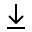MathBin Vector Member

A vector in Euclidean geometry is an object that has magnitude (length) and direction, and can be added to other vectors according to vector algebra. A vector is what is needed to "carry" the point A to the point B; the Latin word vector means "carrier". The magnitude of the vector is the distance between the two points and the direction refers to the direction of displacement from A to B.

Bins in Two Dimensions Bins in Three Dimensions TODO
• Find the component form of the vector given its magnitude and direction angle. v = < vx, vy > = |v| < cos(θ), sin(θ) >,
• Find the resultant vector for two vectors with the same initial point using the Parallelogram Method. For each vector, its magnitude and the angle it makes with the x-axis are given.